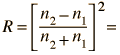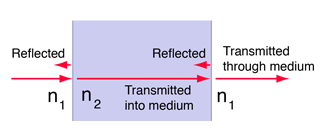# Normal Reflection Coefficient

The reflectivity of light from a surface depends upon the angle of incidence and upon the plane of polarization of the light. The general expression for reflectivity is derivable from Fresnel's Equations. For purposes such as the calculation of reflection losses from optical instruments, it is usually sufficient to have the reflectivity at normal incidence. This normal incidence reflectivity is dependent upon the indices of refraction of the two media.

 For light from a medium of index n1 =
 normally incident upon a medium of index n2 =
 the reflectivity is= %

The reflection coefficients can be used to calculate the amount of light transmitted through a medium if the only losses are reflection losses.

 Reflected from first surface: % Transmitted into medium: % Reflected internally from second surface: % Transmitted through medium: %This calculation of the transmission is not exactly correct since it neglects the contribution from multiple internal reflections in the medium. Since this contribution is proportional to the square and higher powers of the reflection coefficient, it can often be neglected. The reflection loss at a single surface may seem small, but with multiple lenses, it becomes an unacceptable loss. Anti-reflection coatings are used to diminish the losses.

 Light absorption and reflection by opaque objects
 Reflection at other than normal incidence
Index

Reflection concepts

 HyperPhysics***** Light and Vision R Nave
Go Back